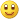## The first Fibonacci Number that is zeroless-pandigital at both ends

Here is another Project Euler problem – this time a little-bit more challenging:

 “The Fibonacci sequence is defined by the recurrence relation: Fn = Fn1 + Fn2 , where F1 = 1 and F2 = 1. It turns out that F541  , which contains 113 digits, is the first Fibonacci number for which the last nine digits are 1-9 pandigital (contain all the digits 1 to 9, but not necessarily in order). And F2749  , which contains 575 digits, is the first Fibonacci number for which the first nine digits are 1-9 pandigital. Given that Fk is the first Fibonacci number for which the first nine digits AND the last nine digits are 1-9 pandigital, find k.”

Does this sound XSLT-ish? No, not at all.

This solution:



has the following properties:

• Execution time:                 34.6 sec.
• LOC:                                       54 (~ 40 express the solution)
• Time to write the code:  ~15 min.
• Uses library:                       FXSL (the f:fibo() function for generating Fibonacci numbers)

The index of the first Fibonacci number with the required properties is more than 300 thousand and the number itself has more than 68 thousand digits.

Not satisfied with the speed of this solution? Want even faster? Then here’s something you may consider:



We have added a a couple of lines of code and look what we have now:

• Execution time:                     0.555 sec.
• LOC:                                        55 (~ 43 express the solution)
• Time to write the code:  ~10 min.

As witnessed in the Project Euler thread for this problem (one must have provided the correct answer in order to access this thread), many solutions took longer: from 3.3 seconds, to 30-40 minutes, to couple of hours.

One Python programmer even waited for the end of his brute-force solution … 12 daysThis entry was posted in Project Euler. Bookmark the permalink.

### 8 Responses to The first Fibonacci Number that is zeroless-pandigital at both ends

1.Khach says:

Dmitri, could you please explain either of these solutions?

•Dimitre Novatchev says:

Khach, What is it that you don’t understand in the first solution? It must be self-explanatory.

•Khach says:

Dmitri,
About the first solution. What the general algorithm of the calculation implemented in the stylesheet and namely what does the second xsl:sequence element? In fact I am not experienced in computational usage of XSLT but would like to gain some experience. Also there is an imported stylesheet ../../../f/func-Fibonacci.xsl. Where is it, or maybe it is the same stylesheet itself?
About the second solution. This seems more elegant but, again, I do not grasp the algorithm.

2.Dimitre Novatchev says:

func-Fibonacci.xsl is part of FXSL. In the post there is already a link to its source — on the smae line that says: “Uses library:: FXSL (the f:fibo() function for generating Fibonacci numbers)” . One can download the latest version of FXSL from its sourceforge CVS.

As for “the second xsl:sequence”, I guess you mean this:

This produces true() exactly when the string representation of \$pN contains all digits from 1 to 9 (in any order.

The XPath translate(\$s1, \$s2, \$s3) function replaces any character in S1 that is also found in \$s2 with its (of \$s2) same-position character in \$s3. When \$s3 is the empty string, then the result is the string \$s1 in which all occurences of characters in \$s2 are deleted.

In this concrete case, if \$s doesn’t contain all digits from 1 to 9, then some digit in the first argument of translate() “123456789” will remain undeleted in the result. Because a non-empty string is automatically converted to the boolean true(), in this case not(translate(’123456789′, \$s, ”)) returns false().

The f:isPandigital19() function is used to check if a number contains all digits 123456789 or not. When passed (as in all cases in this code) a 9-digit number, if it returns true() then this number is zeroless-pandigital.

•Dimitre Novatchev says:

To continue my answer, we first verify that the number is zeroless-pandigital at the end, only then we verify if it is zeroless-pandigital at the head. If both the end and head are zeroless-pandigital — this is our result. If not, then we need to do the same check on the next Fibonacci number.

•Khach says:

Thank you for your explanation. I have to think over it some amount of time.
Is the algorithm of the second stylesheet the same?

3.Dimitre Novatchev says:

No.

•Khach says:

Thank you.